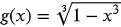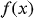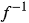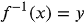1
66
views
32a
Problem

# Problem 32a

## Chapter 1: Functions and ModelsTextbook ExpertVerified Tutor
18 Oct 2021

#### Given information

The function is provided below.#### Step-by-step explanation

Step 1.

Apply the concept to find inverse of a function

First step is to rewriteas y.

Second step is to solve the equation obtained in terms of y for x.

The last step is to interchange x and y to express the functionas a function of x.

The function obtained is the inverse of the function.